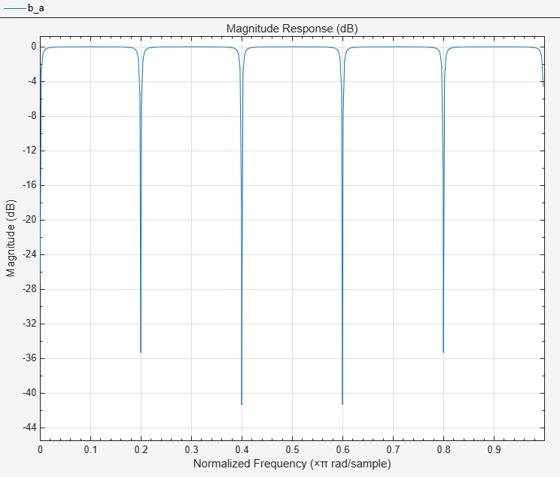# iircomb

IIR comb notch or peak filter

## Syntax

``[num,den] = iircomb(n,bw)``
``[num,den] = iircomb(n,bw,ab)``
``[num,den] = iircomb(___,type)``

## Description

````[num,den] = iircomb(n,bw)` returns a digital notching filter with order `n` and with the width of the filter notch at `-3` dB set to `bw`, the filter bandwidth.For the notching filter, the transfer function takes the form:$H\left(z\right)=b\frac{1-{z}^{-n}}{1-\alpha {z}^{-n}}$where α and b are positive scalars and n is the filter order or the number of notches in the filter minus 1.```
````[num,den] = iircomb(n,bw,ab)` returns a digital notching filter whose bandwidth, `bw`, is specified at a level of –`ab` decibels.```
````[num,den] = iircomb(___,type)` returns either a digital notch filter or a digital peaking filter as specified by `type`.The transfer function for peaking filters is:$H\left(z\right)=b\frac{1-{z}^{-n}}{1+a{z}^{-n}}$```

## Examples

collapse all

Design and plot an IIR notch filter with 11 notches (equal to filter order plus 1) that removes a 60 Hz tone (f0) from a signal at 600 Hz(fs). For this example, set the Q factor for the filter to 35 and use it to specify the filter bandwidth.

```fs = 600; fo = 60; q = 35; bw = (fo/(fs/2))/q; [b,a] = iircomb(fs/fo,bw,'notch'); % Note type flag 'notch'```

Use the Filter Visualization Tool (fvt`ool`) to generate the plot showing the filter notches.

`fvtool(b,a);`Note that the notches are evenly spaced and one falls at exactly 60 Hz.

## Input Arguments

collapse all

Order of the digital filter, specified as a positive integer. `n` also defines the number of notches (or peaks) in the filter across the frequency range 0 to 2π. The number of notches (or peaks) equals `n`+1.

Data Types: `single` | `double`

Bandwidth of the filter at a level of –`ab` decibels, specified as a positive scalar in the range `(0,1)`.

The quality factor (Q factor) q for the filter is related to the filter bandwidth by q = ω0/bw where ω0 is the frequency to remove from the signal.

Data Types: `single` | `double`

Magnitude response at the filter bandwidth `bw`, specified as a scalar in dB. You can include the optional input argument ab to specify the magnitude response at a level other than the default `-3` dB, for example, -6 dB or 0 dB. If you do not include the argument ab, the function sets the magnitude response at the default `-3` dB level.

Data Types: `single` | `double`

Type of the digital filter, specified as either:

• `'notch'` — Design an IIR notch filter. Notch filters attenuate the response at the specified frequencies. This is the default type. When you omit the `type` input argument, `iircomb` function returns a notch filter.

• `'peak'` — Design an IIR peaking filter. Peaking filters boost the signal at the specified frequencies.

## Output Arguments

collapse all

Numerator coefficients of the IIR filter transfer function, returned as a real-valued row vector. For an nth-order filter, the number of elements in the row vector is n+1.

Data Types: `single` | `double`

Denominator coefficients of the IIR filter transfer function, returned as a real-valued row vector. For an nth-order filter, the number of elements in the row vector is n+1.

Data Types: `single` | `double`

 Orfanidis, S.J., Introduction to Signal Processing, Englewood Cliffs, New Jersey: Prentice-Hall, 1996.

Watch now